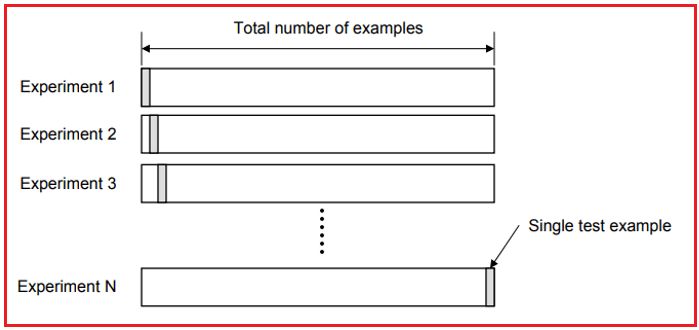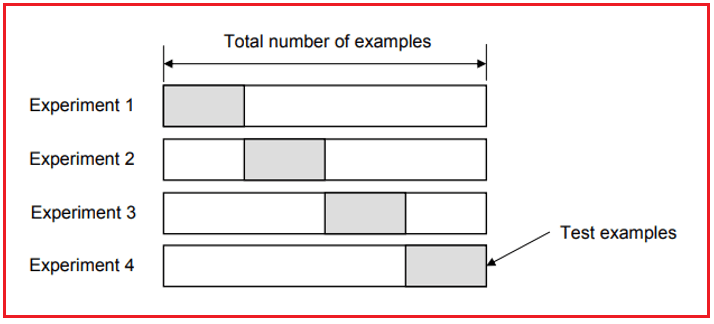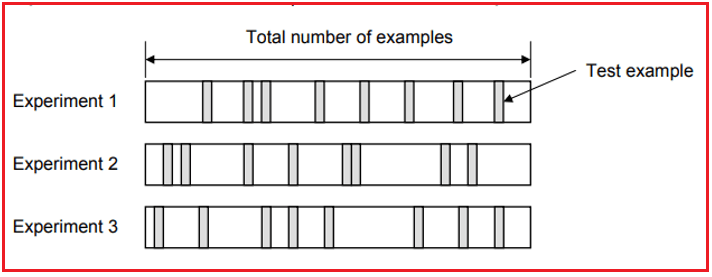# Model Building and Validation in Machine Learning

## Model Building and Validation in Machine Learning

In this article, I am going to discuss Model Building and Validation in Machine Learning with Examples. Please read our previous article where we discussed Univariate, Bivariate, and Multicollinearity Analysis in Machine Learning with Examples.

##### Model Building in Machine Learning

Splitting data into two groups, such as a ‘training set’ and a ‘testing set,’ at the ratio of 80:20 or 70:30, is required for building an ML model.

In machine learning, we either design a classification (if Y is qualitative) or regression (if Y is quantitative) model based on the data type (qualitative or quantitative) of the target variable (often referred to as the Y variable).

Algorithms for machine learning can be divided into three categories:

1. Supervised learning establishes the mathematical link between input X and output Y variables. The labeled data that are used for model building in an effort to learn how to predict the output from the input is made up of such X, and Y pairs.
2. Unsupervised learning is a type of machine learning that uses only the X variables as input. These unlabeled X variables are used by the learning algorithm to model the data’s fundamental structure.
3. Reinforcement learning is a type of machine learning that determines the next course of action by learning via trial and error in order to maximize the reward.
##### Model Validation in Machine Learning

Model validation is the process of evaluating a trained model against a testing data set in machine learning. The training set is inferred from the testing data set, which is a separate chunk of a similar data set. The main purpose of using the testing data set is to see how well a prepared model can speculate. After the model has been trained, it is validated. Model validation, like model training, aims to find the best model with the best execution.

It’s also crucial to choose the right validation method to ensure the accuracy and biases of the validation method. You may not need approval if the data volume is large enough to talk to a large number of people. There are multiple Model Validation techniques. Let’s have a look at them one by one –

##### Leave One Out Cross Validation Method –

All data except one record is used for training in this validation techniques machine learning, and that one record is only used for testing afterward. And if there are N records, the procedure is repeated N times, with the added benefit of being able to use the whole data set for training and testing.This strategy, however, is quite costly because it typically necessitates the creation of a large number of models equivalent to the size of the training data.

The error rate of the model is nearly the average of the error rate of each repetition using this technique. This method provides a good evaluation, although it appears to be highly expensive to compute at first glance.

##### K-Fold Cross Validation Method

According to major AI companies, cross-validation is an important technique for ML model validation that involves training several ML models on subsets of the available input data and evaluating them on the corresponding subset of the data.

This method is intended to detect overfitting or oscillations in the training data that the model selects and learns as concepts. K-fold validation, in which the cross-validation process is performed multiple times with varied divides of the sample data into K-parts, is a more rigorous technique of cross-validation.##### Random Subsampling Validation Method

This technique is frequently used by companies that provide ML algorithm validation services to evaluate the models. Data is randomly partitioned into disjoint training and test sets several times using this method, which implies that multiple sets of data are randomly selected from the dataset and concatenated to form a test dataset, while the remaining data forms the training dataset.The accuracies gained from each partition are averaged, and the model’s error rate is the average of each iteration’s error rate. The random subsampling method has the advantage of being able to be repeated indefinitely.